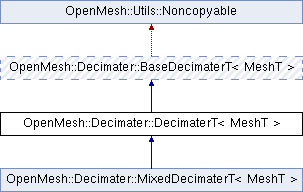Developer Documentation
OpenMesh::Decimater::DecimaterT< MeshT > Class Template Reference

`#include <libs_required/OpenMesh/src/OpenMesh/Tools/Decimater/DecimaterT.hh>`

Inheritance diagram for OpenMesh::Decimater::DecimaterT< MeshT >:## Classes

class  HeapInterface
Heap interface. More...

## Public Types

typedef DecimaterT< MeshT > Self

typedef MeshT Mesh

typedef CollapseInfoT< MeshT > CollapseInfo

typedef ModBaseT< MeshT > Module

typedef std::vector< Module * > ModuleList

typedef ModuleList::iterator ModuleListIterator

typedef Mesh::VertexHandle VertexHandle

typedef Mesh::HalfedgeHandle HalfedgeHandle

typedef Utils::HeapT< VertexHandle, HeapInterfaceDeciHeapPublic Types inherited from OpenMesh::Decimater::BaseDecimaterT< MeshT >
typedef BaseDecimaterT< MeshT > Self

typedef MeshT Mesh

typedef CollapseInfoT< MeshT > CollapseInfo

typedef ModBaseT< MeshT > Module

typedef std::vector< Module * > ModuleList

typedef ModuleList::iterator ModuleListIterator

## Public Member Functions

DecimaterT (Mesh &_mesh)
Constructor.

~DecimaterT ()
Destructor.

size_t decimate (size_t _n_collapses=0)
Perform a number of collapses on the mesh. More...

size_t decimate_to (size_t _n_vertices)
Decimate the mesh to a desired target vertex complexity. More...

size_t decimate_to_faces (size_t _n_vertices=0, size_t _n_faces=0)
Attempts to decimate the mesh until a desired vertex or face complexity is achieved. More...Public Member Functions inherited from OpenMesh::Decimater::BaseDecimaterT< MeshT >
BaseDecimaterT (Mesh &_mesh)

bool initialize ()

bool is_initialized () const
Returns whether decimater has been successfully initialized.

void info (std::ostream &_os)
Print information about modules to _os.

void set_observer (Observer *_o)

Observerobserver ()
Get current observer of a decimater.

Mesh & mesh ()
access mesh. used in modules.

template<typename _Module >
bool add (ModHandleT< _Module > &_mh)

template<typename _Module >
bool remove (ModHandleT< _Module > &_mh)
remove module

template<typename Module >
Modulemodule (ModHandleT< Module > &_mh)
get module referenced by handle _mh

## Private Member Functions

void heap_vertex (VertexHandle _vh)
Insert vertex in heap.

## Private Attributes

Mesh & mesh_

std::auto_ptr< DeciHeapheap_

VPropHandleT< HalfedgeHandle > collapse_target_

VPropHandleT< float > priority_

VPropHandleT< int > heap_position_Protected Member Functions inherited from OpenMesh::Decimater::BaseDecimaterT< MeshT >
bool notify_observer (size_t _n_collapses)
returns false, if abort requested by observer

void set_uninitialized ()
Reset the initialized flag, and clear the bmodules_ and cmodule_.

void update_modules (CollapseInfo &_ci)

bool is_collapse_legal (const CollapseInfo &_ci)

float collapse_priority (const CollapseInfo &_ci)
Calculate priority of an halfedge collapse (using the modules)

void preprocess_collapse (CollapseInfo &_ci)
Pre-process a collapse.

void postprocess_collapse (CollapseInfo &_ci)
Post-process a collapse.

void set_error_tolerance_factor (double _factor)

void reset ()

## Detailed Description

### template<typename MeshT> class OpenMesh::Decimater::DecimaterT< MeshT >

Decimater framework.

BaseModT, Mesh Decimation Framework

Definition at line 83 of file DecimaterT.hh.

## Member Function Documentation

template<class Mesh >
 size_t OpenMesh::Decimater::DecimaterT< Mesh >::decimate ( size_t _n_collapses = `0` )

Perform a number of collapses on the mesh.

Parameters
 _n_collapses Desired number of collapses. If zero (default), attempt to do as many collapses as possible.
Returns
Number of collapses that were actually performed.
Note
This operation only marks the removed mesh elements for deletion. In order to actually remove the decimated elements from the mesh, a subsequent call to ArrayKernel::garbage_collection() is required.

Definition at line 156 of file DecimaterT.cc.

template<typename MeshT >
 size_t OpenMesh::Decimater::DecimaterT< MeshT >::decimate_to ( size_t _n_vertices )
inline

Decimate the mesh to a desired target vertex complexity.

Parameters
 _n_vertices Target complexity, i.e. desired number of remaining vertices after decimation.
Returns
Number of collapses that were actually performed.
Note
This operation only marks the removed mesh elements for deletion. In order to actually remove the decimated elements from the mesh, a subsequent call to ArrayKernel::garbage_collection() is required.

Definition at line 124 of file DecimaterT.hh.

template<class Mesh >
 size_t OpenMesh::Decimater::DecimaterT< Mesh >::decimate_to_faces ( size_t _n_vertices = `0`, size_t _n_faces = `0` )

Attempts to decimate the mesh until a desired vertex or face complexity is achieved.

Parameters
 _n_vertices Target vertex complexity. _n_faces Target face complexity.
Returns
Number of collapses that were actually performed.
Note
Decimation stops as soon as either one of the two complexity bounds is satisfied.
This operation only marks the removed mesh elements for deletion. In order to actually remove the decimated elements from the mesh, a subsequent call to ArrayKernel::garbage_collection() is required.

Definition at line 260 of file DecimaterT.cc.

The documentation for this class was generated from the following files:
• libs_required/OpenMesh/src/OpenMesh/Tools/Decimater/DecimaterT.hh
• libs_required/OpenMesh/src/OpenMesh/Tools/Decimater/DecimaterT.cc